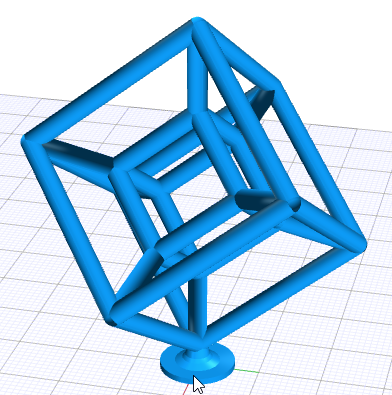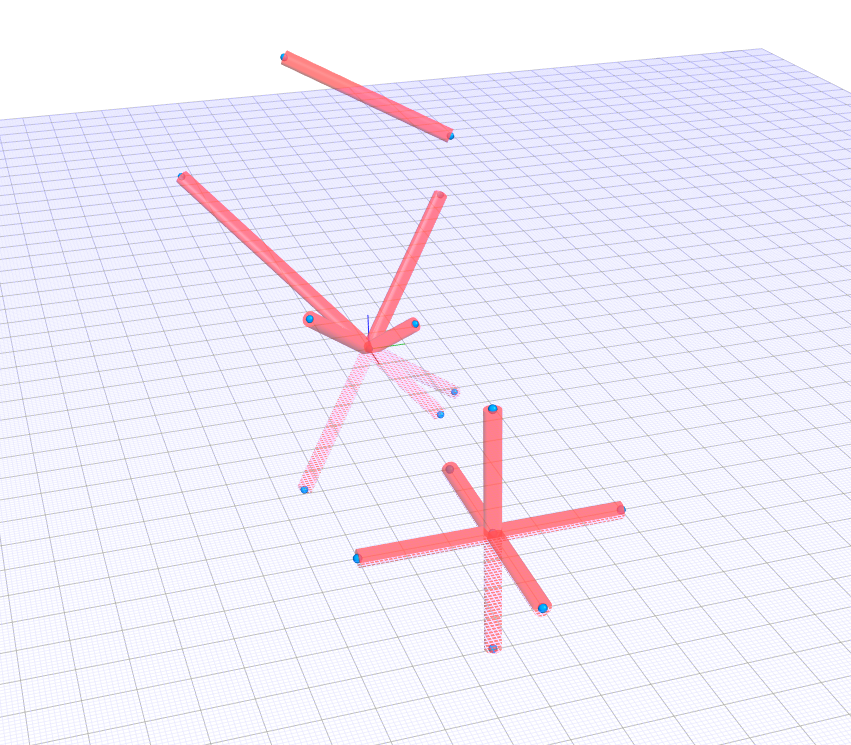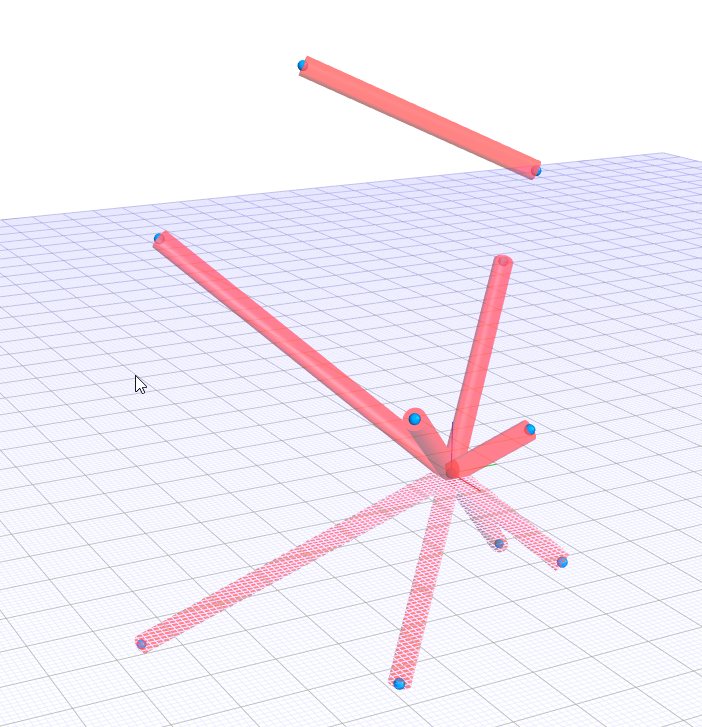# Cylinder between points?

• At the risk of being a pain in the neck (or choose other part of the body) with all my current enquiries, I have another one. In V1, and in CSG, we had the (for me) very useful functionality of being able to construct a cylinder between two given points. This does not seem to exist in V2. Now I know that a cylinder between two points can be constructed first by determining a height as the Euclidean distance between the points, and width, and then using appropriate rotations and a translation to move it into its place. But this is a non-trivial task (how would you construct a cylinder between (-2,3,5) and (-4,-1,7) for example?) which would be rendered much easier by being able to construct a cylinder between two points. Will this be added, or is this one of those libraries to be added by users to make up any loss of functionality from V1 and CSG? (We expect REMOVED functionality to be supported via small user libraries.) If so, I'd better get to work!

Thanks,
Alasdair

• I have sth with this function• @Alasdair-McAndrew
I tried to find code that does this exactly, but failed. So I took some time try different combinations of atan acos asin until I got somewhat working solution. Then I added fixes for special cases that break the inital code.

based on your suggestion I tried some values (`dx=0`, `dy=0`, `dz=0`) and found a case that did not work: `(dx==0 && dy == 0)`.
I have added an exception that now fixes taht edge case.

(I am proud I made this work somehow, so if you find another edge case that does not work, let me know)

new version with fix:

``````function cylinderFromTo(p1,p2, radius){
const sqr = x=>x*x

let dx = p2 - p1
let dy = p2 - p1
let dz = p2 - p1

let height = Math.sqrt( sqr(dx) + sqr(dy) + sqr(dz))

if(dx || dy){
let dxy = Math.sqrt( sqr(dx) + sqr(dy))

let ay = Math.atan(dxy/dz) *(dx < 0 ? -1:1)
let az = Math.atan(dy/dx)
let ax = dz < 0 ? -Math.PI:0
obj = transforms.rotate([ax,ay,az], obj)
}

let mid = [p1+dx/2,p1+dy/2,p1+dz/2]

return translate(mid, obj)
}
``````

the code now works for all of these:

``````  testCylinderFromTo([-20,30,50],[-40,-10,70]);
testCylinderFromTo([0,0,0],[10,10,10])
testCylinderFromTo([0,0,0],[20,-20,20])
testCylinderFromTo([0,0,0],[-30,30,30])
testCylinderFromTo([0,0,0],[-40,-40,40])

testCylinderFromTo([0,0,0],[15,15,-15])
testCylinderFromTo([0,0,0],[25,-25,-25])
testCylinderFromTo([0,0,0],[-35,35,-35])

testCylinderFromTo([100,0,0],[100,0,30])
testCylinderFromTo([100,0,0],[100,0,-30])
testCylinderFromTo([100,0,0],[100,30,0])
testCylinderFromTo([100,0,0],[100,-30,0])
testCylinderFromTo([100,0,0],[130,0,0])
testCylinderFromTo([100,0,0],[70,0,0])
``````

screen:• @hrgdavor That looks terrific! I'm working on a similar one, but trying to see what happens if two of the coordinates are equal, or zero. That is, if the x-coordinates of p1 and p2 are equal, or the y-coordinates, etc. Or if some of them are zeros. For example, in your computations of ay and az, you're using an inverse tangent, but what happens if either dx = 0, or dz = 0?

I decided to first translate p1 and p2 to 0 and p2-p1 or p1-p2, depending on which had a positive z-coordinate. Then we are simply rotating things in the z>=0 half-space, which reduces everything to two rotations (as you have).

But I do like the simplicity and elegance of your code: clearly you're a more advanced JavaScript programmer than me. Did you work all this out from scratch, or are you taking another program and rewriting it?

The other possible issue is that the result should be independent of the order of p1 and p2: it may be that your function does that automatically, but I found it tricky with mine.

Thank you very much indeed.

• I thought it would be fun to make such function.

It is a product of using atan acos asin until I got something and then guessing some more until it worked. It seems to be working, though I actually dont understand it completely``````function cylinderFromTo(p1,p2, radius){
const sqr = x=>x*x
let dx = p2 - p1
let dy = p2 - p1
let dz = p2 - p1
let dxy = Math.sqrt( sqr(dx) + sqr(dy))
let height = Math.sqrt( sqr(dx) + sqr(dy) + sqr(dz))

let ay = Math.atan(dxy/dz) *(dx < 0 ? -1:1)
let az = Math.atan(dy/dx)
let ax = dz < 0 ? -Math.PI:0
let mid = [p1+dx/2,p1+dy/2,p1+dz/2]
return translate(mid, rotate([ax,ay,az], obj))
}

``````

I used these to test visually

``````function main(){
ret = [];

function testCylinderFromTo(p1,p2){
ret = [ ...ret,
translate(p1,sphere({size:1})),
translate(p2,sphere({size:1})),
];
}

testCylinderFromTo([-20,30,50],[-40,-10,70]);
testCylinderFromTo([0,0,0],[10,10,10])
testCylinderFromTo([0,0,0],[20,-20,20])
testCylinderFromTo([0,0,0],[-30,30,30])
testCylinderFromTo([0,0,0],[-40,-40,40])

testCylinderFromTo([0,0,0],[15,15,-15])
testCylinderFromTo([0,0,0],[25,-25,-25])
testCylinderFromTo([0,0,0],[-35,35,-35])
testCylinderFromTo([0,0,0],[-45,-45,-45])

return ret;
}
``````• @Alasdair-McAndrew Hopefully someone will provide the code, but oblique cylinders can easily be created using extrudeLinear().